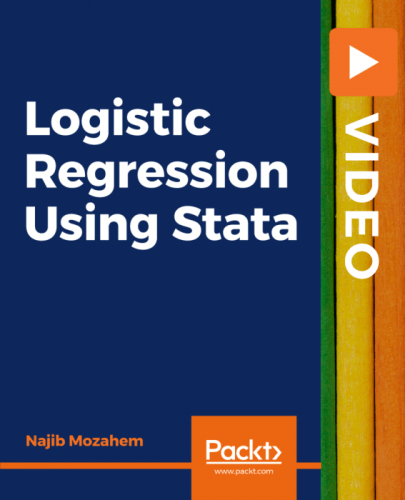Packt - Logistic Regression Using Stata-XQZT
English | Size: 1.15 GB
Category: Tutorial

Stata is one of the leading statistical software packages widely used in different fields. This course is divided into two parts The first part covers the theory behind logistic regression, and the second part enables you to apply the theory to practical scenarios using Stata.
Starting with an introduction to contingency tables, you ll learn how to interpret the odds and calculate the odds ratios. You ll then understand when and how to use the logistic regression technique. The course covers topics such as model building prediction, and assessment of model fit. Additionally, it will help you get to grips with diagnostics by explaining the concept of residuals and influential observations. As you advance, you ll be taken through a real-world project to understand and implement various commands.
By the end of this course, you ll have all the knowledge you need to apply logistic regression for descriptive statistics. All codes and supporting files are available